• 第一产业包括一切直接从地球开采资源的行业；
• 第二产业包括所有进行加工的行业；
• 第三产业指一切提供服务的行业；
• 第四产业指进行学术研究探索，维持秩序的工作，服务对象为社会。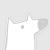0+0+0+0+0+0+0+0+0+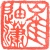0+0+0+0+0+0+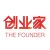0+0+0+0+0+0+0+0+0+0+0+0+0+0+0+0+0+0+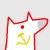0+0+0+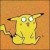0+0+0+0+0+0+0+0+0+
Top